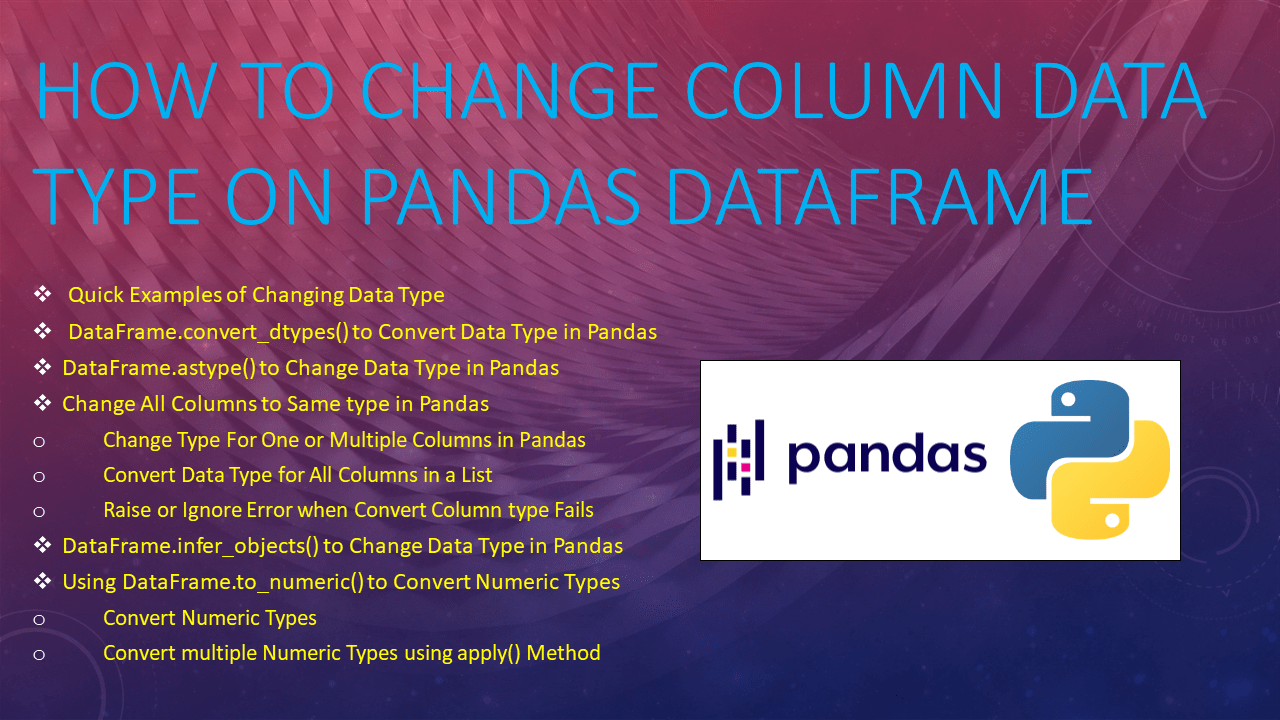# Different Ways to Change Data Type in pandas

While working in Pandas DataFrame or any table-like data structures we are often required to chang the data type(dtype) of a column also called type casting, for example, convert from int to string, string to int e.t.c, In pandas, you can do this by using several methods like `astype()`, `to_numeric()`, `covert_dttypes()`, `infer_objects()` and e.t.c. In this article, I will explain different examples of how to change or convert the data type in Pandas DataFrame – convert all columns to a specific type, convert single or multiple column types – convert to numeric types e.t.c.

## 1. Quick Examples of Changing Data Type

Below are some quick examples of converting column data type on Pandas DataFrame.

``````
# Quick Examples of Converting Data Types in Pandas
df2=df.convert_dtypes()
df = df.astype(str)
df = df.astype({"Fee": int, "Discount": float})
df = df.astype({"Courses": int},errors='ignore')
df = df.infer_objects()
df['Fee'] = pd.to_numeric(df['Fee'])
df[['Fee', 'Discount']] =df [['Fee', 'Discount']].apply(pd.to_numeric)
``````

Now let’s see with an example. first, create a Pandas DataFrame with columns names `Courses`, `Fee`, `Duration`, `Discount`.

``````
import pandas as pd
technologies = {
'Fee' :[20000,25000,26000,22000,24000,21000,22000],
'Duration ':['30day','40days','35days', '40days','60days','50days','55days'],
'Discount':[11.8,23.7,13.4,15.7,12.5,25.4,18.4]
}
df = pd.DataFrame(technologies)
print(df.dtypes)
``````

Yields below output.

``````
Courses       object
Fee            int64
Duration      object
Discount     float64
``````

## 2. DataFrame.convert_dtypes() to Convert Data Type in Pandas

`convert_dtypes()` is available in Pandas DataFrame since version 1.0.0, this is the most used method as it automatically converts the column types to best possible types.

Below is the Syntax of the `pandas.DataFrame.convert_dtypes()`.

``````
# Syntax of DataFrame.convert_dtypes
DataFrame.convert_dtypes(infer_objects=True, convert_string=True,
convert_integer=True, convert_boolean=True, convert_floating=True)
``````

Now, let’s see a simple example.

``````
# Convert all types to best possible types
df2=df.convert_dtypes()
print(df2.dtypes)
``````

Yields below output. Note that it converted columns with `object` type to `string` type.

``````
Courses       string
Fee            int64
Duration      string
Discount     float64
``````

## 3. DataFrame.astype() to Change Data Type in Pandas

In pandas DataFrame use `<a href="https://pandas.pydata.org/docs/reference/api/pandas.DataFrame.astype.html#">DataFrame.astype()</a>` to convert one type to another type of single or multiple columns at a time, you can also use it to change all column types to the same type. When you perform astype() on a DataFrame without specifying a column name, it changes all columns to a specific type. To convert a specific column, you need to explicitly specify the column.

Below is the syntax `of pandas.DataFrame.astype()`

``````
# Below is syntax of DataFrame.astype()
DataFrame.astype(dtype, copy=True, errors='raise')
``````

### 3.1 Change All Columns to Same type in Pandas

`df.astype(str)` converts all columns of Pandas DataFrame to `string` type.

``````
# Change All Columns to Same type
df = df.astype(str)
print(df.dtypes)
``````

Yields below output.

``````
Courses      object
Fee          object
Duration     object
Discount     object
dtype: object
``````

### 3.2 Change Type For One or Multiple Columns in Pandas

On `astype()` Specify the param as JSON notation with column name as key and type you wanted to convert as a value to change one or multiple columns. Below example cast DataFrame column `Fee` to `int` type and `Discount` to `float` type.

``````
# Change Type For One or Multiple Columns
df = df.astype({"Fee": int, "Discount": float})
print(df.dtypes)
``````

## 3.3 Convert Data Type for All Columns in a List

Sometimes you may need to convert a list of DataFrame columns to a specific type, you can achieve this in several ways. Below are 3 different ways that coverts columns `Fee` and `Discount` to `float` type.

``````
# Convert Data Type for All Columns in a List
df = pd.DataFrame(technologies)
cols = ['Fee', 'Discount']
df[cols] = df[cols].astype('float')

# By using a loop
for col in ['Fee', 'Discount']:
df[col] = df[col].astype('float')

#By using apply() & astype() together
df[['Fee', 'Discount']].apply(lambda x: x.astype('float'))
``````

### 3.4 Raise or Ignore Error when Convert Column type Fails

By default, when you are trying to change a column to a type that is not supported with the data, Pandas generates an error, in order to ignore error use errors param; this takes either ignore or error as value. In the below example I am converting a column that has string value to int which is not supported hence it generates an error, I used `errors='ignore'` to ignore the error.

``````
#Ignores error
df = df.astype({"Courses": int},errors='ignore')

# Generates error
df = df.astype({"Courses": int},errors='raise')
``````

## 4. DataFrame.infer_objects() to Change Data Type in Pandas

Use `DataFrame.infer_objects()` method to automatically convert object columns to a type of data it holding. It checks the data of each object column and automatically converts it to data type. Note that it converts only object types. For example, if a column with object type is holding int or float types, using infer_object() converts it to respective types.

``````
# Converts object types to possible types
df = pd.DataFrame(technologies)
df = df.infer_objects()
print(df.dtypes)
``````

## 5. Using DataFrame.to_numeric() to Convert Numeric Types

`pandas.DataFrame.to_numeric()` is used to convert columns with non-numeric `dtypes` to the most suitable numeric type.

## 5.1 Convert Numeric Types

The below example just converts `Fee` column to the numeric type.

``````
# Converts feed column to numeric type
df['Fee'] = pd.to_numeric(df['Fee'])
``````

### 5.2 Convert multiple Numeric Types using apply() Method

Use `to_numeric()` along with `DataFrame.apply()` method to convert multiple columns into a numeric type. Below example converts column `Fee` and `Discount` to numeric types.

``````
# Convert Fee and Discount to numeric types
df = pd.DataFrame(technologies)
df[['Fee', 'Discount']] =df [['Fee', 'Discount']].apply(pd.to_numeric)
print(df.dtypes)
``````

### Conclusion

In this article, you have learned how to convert/change all columns of the DataFrame to a specific type, case one or multiple columns and finally converting columns to numeric type using `astype()`, `to_numeric()`, `covert_dttypes()`, `infer_objects()` methods.

Happy Learning !!

### NNK

SparkByExamples.com is a Big Data and Spark examples community page, all examples are simple and easy to understand and well tested in our development environment Read more ..